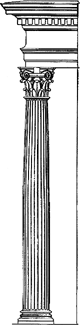# composite order

Also found in: Dictionary, Thesaurus, Wikipedia.
Related to composite order: Corinthian order, Classical orders

## composite order:

see Corinthian orderCorinthian order,
most ornate of the classic orders of architecture. It was also the latest, not arriving at full development until the middle of the 4th cent. B.C. The oldest known example, however, is found in the temple of Apollo at Bassae (c.420 B.C.).
.

## Composite order

One of the five classical orders; a Roman elaboration of the Corinthian order; the acanthus leaves of its capitals are combined with the large volutes of the Ionic order and set on the diagonal in plan view.

## Composite orderComposite order
In Classical architecture, one of the five Classical orders; combines characteristics of both the Corinthian and Ionic orders; similar to the Corinthian order, but much more embellished. The capital consists of volutes borrowed, with modifications, from the Ionic capital; the circle of acanthus leaves applied to the capital is borrowed from the Corinthian capital. See illustration under bases for an example of a base of the Composite order.
McGraw-Hill Dictionary of Architecture and Construction. Copyright © 2003 by McGraw-Hill Companies, Inc.
References in periodicals archive ?
Composite order bilinear groups first appeared in the literature .
As previously mentioned, inputting the security parameter [lambda], the algorithm Q generates composite order bilinear groups (P = [p.sub.1][p.sub.2][p.sub.3], G, [G.sub.T], e).
First, the PKG runs the algorithm G([1.sup.[lambda]]) to produce composite order bilinear groups (p, G, [G.sub.T], e).
Specifically, the anonymity is guaranteed in virtue of the orthogonality of the bilinear map for composite order bilinear groups in the phases of secret key generation and encryption.
The security of our construction relies on three subgroup decision related assumptions on bilinear groups of composite order. They have been used by Lewko and Waters to prove the security of their IBE  and ABE .
Assumption 1 : Given a bilinear group of composite order (N, G, [G.sub.T], [??]) and random [MATHEMATICAL EXPRESSION NOT REPRODUCIBLE IN ASCII], it is hard to distinguish a random element [MATHEMATICAL EXPRESSION NOT REPRODUCIBLE IN ASCII] from a random element [T.sub.2] [member of] Gpi
Assumption 2: Given a bilinear group of composite order (N, G, [G.sub.T], [??]) and random g, [X.sub.1] [member of] [G.sub.p1], [X.sub.2], [Y.sub.2] [member of] [G.sub.p2], [X.sub.3], [Y.sub.3] [member of] [G.sub.p3], it is hard to distinguish a random element [T.sub.1] [member of] G from a random element [MATHEMATICAL EXPRESSION NOT REPRODUCIBLE IN ASCII].
Assumption 3: Given a bilinear group of composite order (N, G, [G.sub.T], [??]) and random [MATHEMATICAL EXPRESSION NOT REPRODUCIBLE IN ASCII], it is hard to distinguish the element [T.sub.1] = [??][(g, g).sup.[alpha]s] from a random element [T.sub.2] [member of] [G.sub.T].
For the efficiency of anonymous HIBE, it is better to use prime order bilinear groups and have constant size ciphertexts than to use composite order bilinear groups and have linear size ciphertexts.
The detailed information of composite order bilinear groups and prime order symmetric bilinear groups is summarized in Table 2.
The detailed information of bilinear groups Bilinear Group Security Group Order [absolute value of G] Composite Order 80 bits 1024 bits 1024 bits Prime Order 80 bits 160 bits 512 bits Bilinear Group [absolute value of G] [T.sub.exp] Composite Order 2048 bits O([rb.sup.2]) Prime Order 1024 bits O([rb.sup.2]) Bilinear Group [T.sub.pair] Composite Order 757 ms Prime Order 25 ms [T.sub.xp] = exponentiation time, [T.sub.exp] = pairing time, r = the size of group, b = the size of G Table 3.
As a result, we're predicting a gain of almost 10% in our composite orders index during 2002.

Site: Follow: Share:
Open / Close